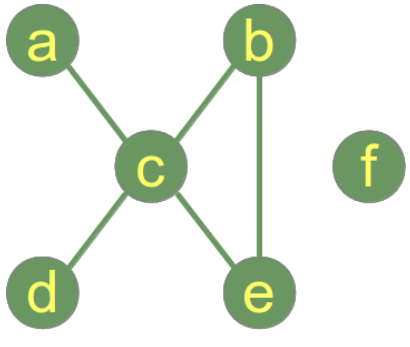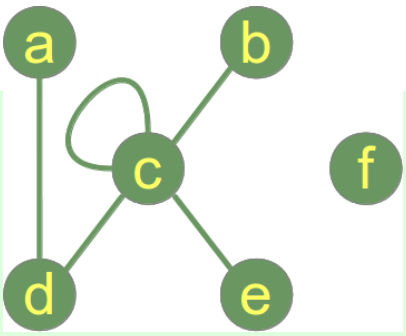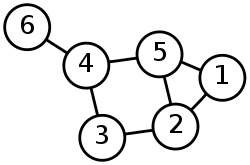# Python Learning Week 4

## Graph Theory and Path Searches in Python

Background

Graph Theory

Graph theory and in particular the graph ADT (abstract data-type) is widely explored and implemented in the field of Computer​​ Science and Mathematics. Consisting of vertices (nodes) and the edges (optionally directed/weighted) that connect them, the data-structure is effectively able to represent and solve many problem domains. One of the most popular areas of algorithm design within this space is the problem of checking for the existence or (shortest) path between two or more vertices in the graph. Properties such as edge weighting and direction are two such factors that the algorithm designer can take into consideration. In this​​ post I will be exploring two of the simpler available algorithms, Depth-First and Breath-First search to achieve the goals highlighted below:

• Find all vertices in a subject vertex connected component.

• Return all available paths between two vertices.

And in the case of BFS, return the shortest path (length measured by number of path edges).

In our illustration, - which is a pictorial representation of a graph, - the node "a" is​​ connected with the node "c", but "a" is not connected with "b". The connecting line between two nodes is called an edge. If the edges between the nodes are undirected, the graph is called an undirected graph. If an edge is directed from one vertex (node)​​ to another, a graph is called a directed graph. An directed edge is called an arc.Python has no built-in data type or class for graphs, but it is easy to implement them in Python. One data type is ideal for representing graphs in Python, i.e. dictionaries.The keys of the dictionary above are the nodes of our graph. The corresponding values are lists with the nodes, which are connecting by an edge. There is no simpler and more elegant way to represent a graph.

An edge can be seen as a 2-tuple with nodes as elements, i.e. ("a","b")

Paths in Graph

We want to find now the shortest path from one node to another node.

Two vertices are adjacent when they are both incident to a common edge.

• Path in an undirected Graph:
A path in an​​ undirected graph is a sequence of vertices P = ( v1, v2, ..., vn )​​ ​​ V x V x ... x V such that vi is adjacent to v{i+1} for 1 ≤ i < n. Such a path P is called a path of length n from v1 to vn.

• Simple Path:
A path with no repeated vertices is called a simple path.

Degree of a Graph

The degree of a vertex v in a graph is the number of edges connecting it, with loops counted twice. The degree of a vertex v is denoted deg(v). The maximum degree of a graph G, denoted by Δ(G), and the minimum degree of a graph, denoted by δ(G), are the maximum and minimum degree of its vertices.

In the graph on the right side, the maximum degree is 5 at vertex c and the minimum degree is 0, i.e the isolated vertex f.Implement the given Graph in Python.​​• ​​ Write​​ a method to output degree of each node within the graph.

• ​​ Write a method to find any path from node 6 to node 1 in given Graph.

• ​​ Modify part 2 to show all possible paths between node 6 to node 1 in Graph.

​​

Check solution here Python Learning Week 4 (Solution)